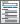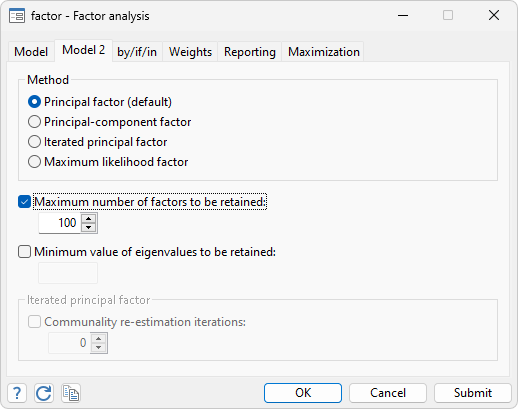Home  /  Products  /  Features  /  Multivariate methods

## Multivariate methods

Use multivariate analyses to evaluate relationships among variables from many different perspectives. Perform multivariate tests of means, or fit multivariate regression and MANOVA models. Explore relationships between two sets of variables, such as aptitude measurements and achievement measurements, using canonical correlation. Examine the number and structure of latent concepts underlying a set of variables using exploratory factor analysis. Or use principal component analysis to find underlying structure or to reduce the number of variables used in a subsequent analysis. Discover groupings of observations in your data using cluster analysis. If you have known groups in your data, describe differences between them using discriminant analysis. And much more.

Factor analysisDiscriminant analysisZellner’s seemingly unrelated regression• Two-step or maximum likelihood estimates
• Linear constraints
• Breusch-Pagan test for independent equations

Multivariate linear regressionProcrustes analysis

Canonical correlationsTetrachoric correlations• Maximum likelihood or noniterative Edwards and Edwards estimator
• Tetrachoric correlation coefficient and standard error
• Exact two-sided significance level
• Complete implementation
• Complete implementation
• Complete implementation

Multivariate tests

Multidimensional scaling

Correspondence analysis

• View and run all postestimation features for your command
• Automatically updated as estimation commands are run
• Display your choice of any two biplot dimensions
• Distinguish groups of data within the biplot
• Display table of biplot coordinates
• Generate new variables containing biplot coordinates

Hotelling’s T-squaredCronbach’s alpha• Interitem correlations or covariances
• Generate summative scale
• Automatically reverse sense of variables